# Normal line and two flat mirrors at right angles

When two plane mirrors are at right angles to each other a ray of light reflects from one mirror into the other and back out again.

The outgoing reflected ray is always parallel to the incident ray

Using the law of reflection and geometry we can show that the outgoing reflected ray is always parallel to the incident ray.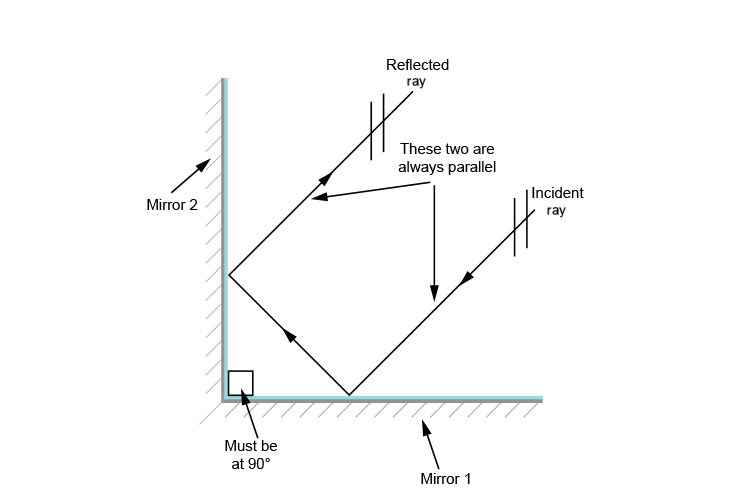We can prove this as follows:

First of all put the normals in where the ray touches the mirrors and extend them so they meet each other.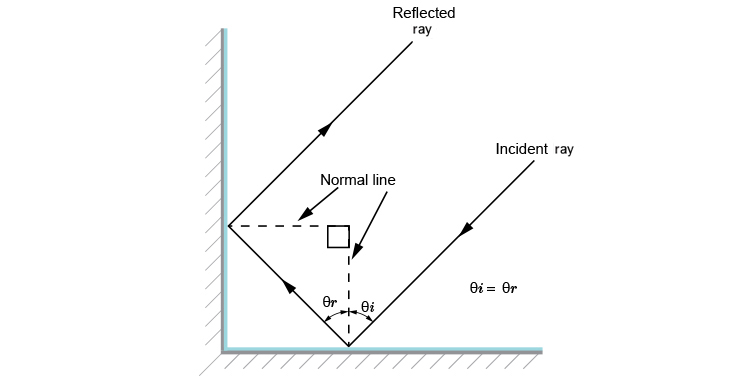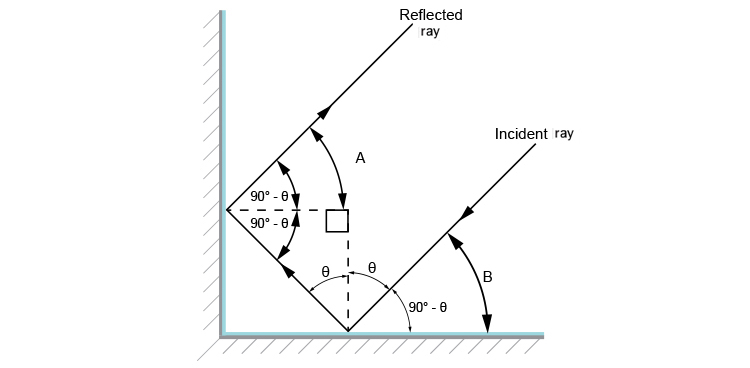We can see that A and B equal 90^@-(m\i\n\u\s)theta.

No matter what angle theta is the incident ray and reflected ray will be parallel.

Let's show you with two different angles.

Example 1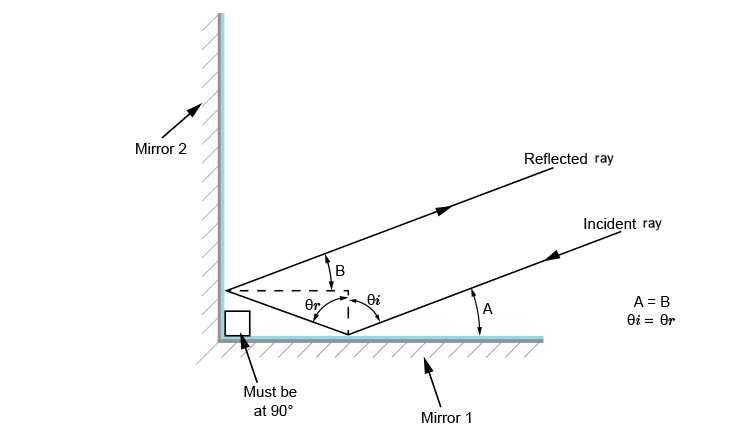Angle A equals angle B and equal 90^@-(m\i\n\u\s)theta

Example 2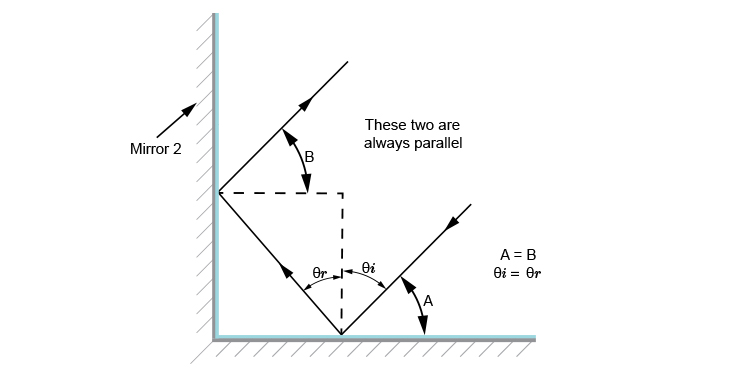Angle A equals angle B and equal 90^@-(m\i\n\u\s)theta